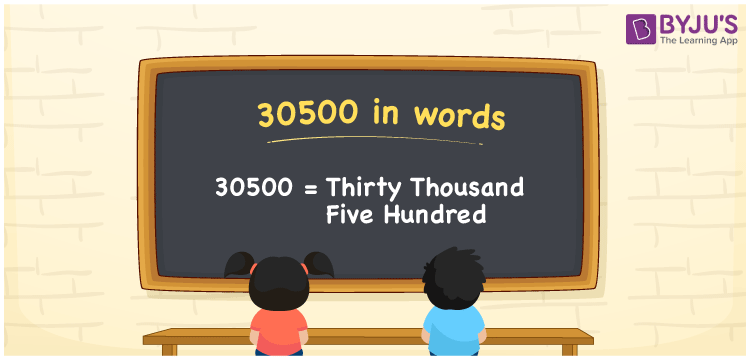# 30500 in Words

30500 in words is Thirty thousand Five hundred. Thus, whenever we use the number 30500, we can pronounce it as Thirty thousand five hundred. We can convert a number to words with the help of a place value chart. In this article, you can understand how to write the cardinal number 30500 in words.

 30500 in words Thirty thousand Five hundred Thirty thousand Five hundred in Numbers 30500

## 30500 in English words

Generally, we can express numbers in words using the English alphabet. So, we can read 30500 in English as “Thirty thousand Five hundred”.## How to Write 30500 in Words?

Let us create the place value chart with five columns since 30500 is a five-digit number.

 Ten thousands Thousands Hundreds Tens Ones 3 0 5 0 0

From the above table, we can expand the numbers as:

3 × Ten thousand + 0 × Thousand + 5 × Hundred + 0 × Ten + 0 × One

= 3 × 10000 + 0 × 1000 + 5 × 100 + 0 × 10 + 0 × 1

= 30000 + 500

= Thirty thousand + Five hundred

= Thirty thousand Five hundred

Hence, 30500 in words = Thirty thousand Five hundred.

### Facts About the Number 30500

30500 is a natural number that is the successor of 30499 and the predecessor of 30501.

30500 in words – Thirty thousand Five hundred

Is 30500 an odd number? – No

Is 30500 an even number? – Yes

Is 30500 a perfect square number? – No

Is 30500 a perfect cube number? – No

Is 30500 a prime number? – No

Is 30500 a composite number? – Yes

## Frequently Asked Questions on 30500 in Words

Q1

### How do you write 30500 in English words?

We can write 30500 in English words as Thirty thousand Five hundred.
Q2

### How do you write 30500 on a cheque?

On a cheque, 30500 can be written as Thirty thousand Five hundred rupees only.
Q3

### What is the value of thirty thousand and five hundred minus thirty thousand?

Thirty thousand and five hundred minus Thirty thousand = 30500 – 30000 = 500 The value of thirty thousand and five hundred minus thirty thousand is five hundred.